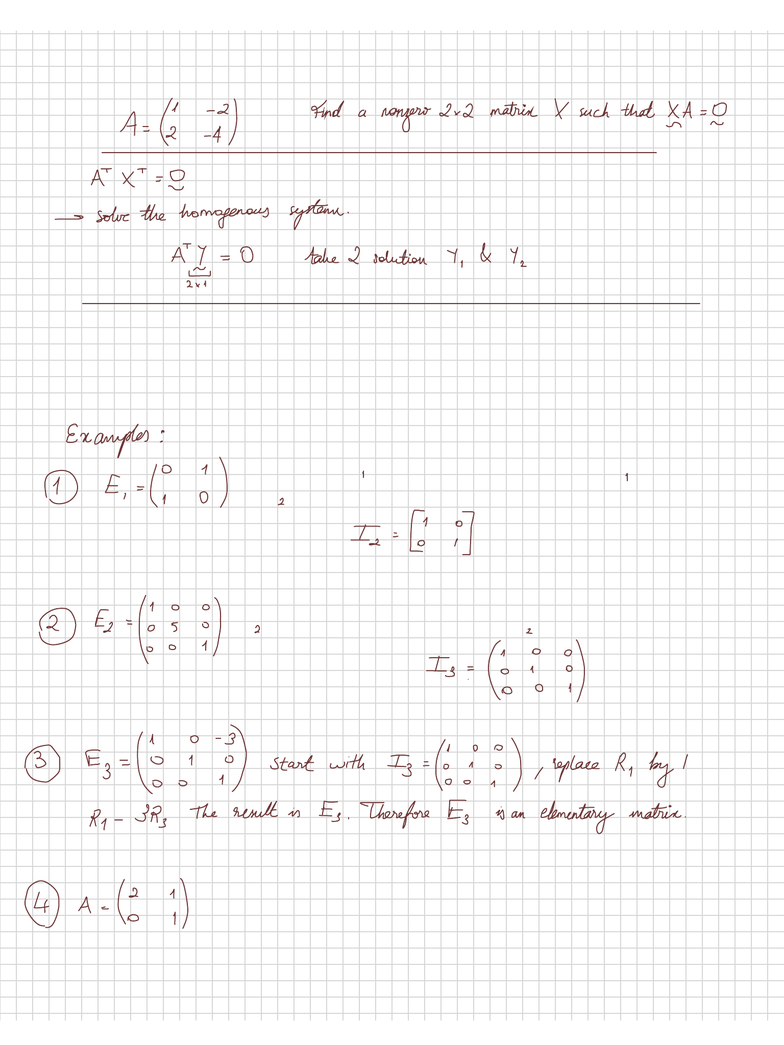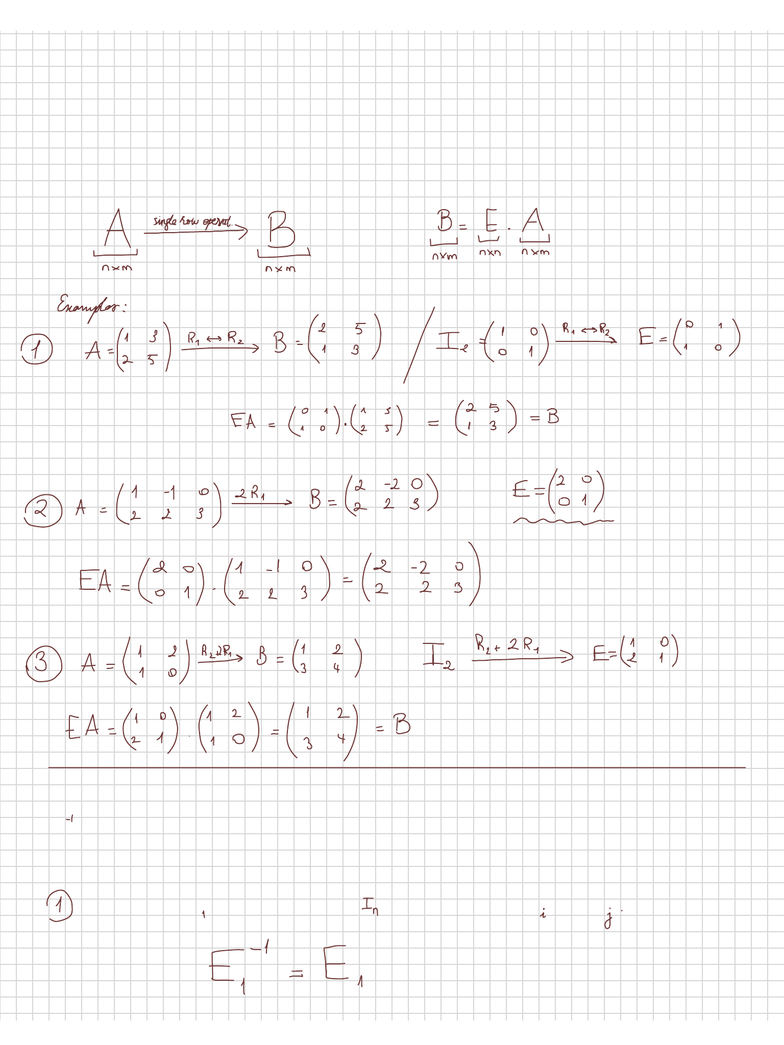Class Notes (1,100,000)
CA (650,000)
McGill (40,000)
MATH (200)
MATH 133 (300)
Lecture

# MATH 133 Lecture Notes - Elementary Matrix, Identity Matrix, Invertible Matrix

Department
Mathematics & Statistics (Sci)
Course Code
MATH 133
Professor
Djivede Kelome

This preview shows pages 1-2. to view the full 6 pages of the document.Math 133
Exercise:
Elementary matrices
A nxn elementary matrix is a matrix obtained by performing a
single elementary row operation ont he nxn identity matrix (In)
Note that E is obtained by exchanging R and
R starting with
E is obtained by multiplying R by 5 starting with
A is not an elementary matrix

Only pages 1-2 are available for preview. Some parts have been intentionally blurred.Theorem:!
let A be a nxm matrix!
Performing a single row operation on A is equivalent to multiplying
A from the left by an elementary matrix.
E; Eis obtained by performing the same row operation
Theorem: if E is an elementary matrix then E is invertible and actually
E is also an elementary matrix.
Suppose that E is obtained from by exchanging R and R